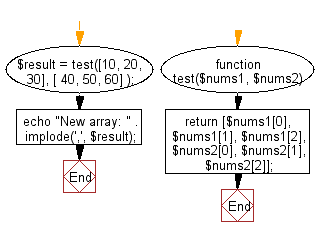﻿ PHP Exercises: Create a new array from two give array of integers, each length 3 - w3resource# PHP Exercises: Create a new array from two give array of integers, each length 3

## PHP Basic Algorithm: Exercise-101 with Solution

Write a PHP program to create a new array from two give array of integers, each length 3.

Sample Solution:

PHP Code :

``````<?php
function test(\$nums1, \$nums2)
{

return [\$nums1, \$nums1, \$nums1, \$nums2, \$nums2, \$nums2];
}

\$result = test([10, 20, 30], [ 40, 50, 60] );
echo "New array: " . implode(',', \$result);
``````

Sample Output:

```New array: 10,20,30,40,50,60
```

Flowchart:PHP Code Editor:

What is the difficulty level of this exercise?

﻿

## PHP: Tips of the Day

\$_REQUEST: This SuperGlobal Variable is used to collect data submitted by a HTML Form

Example:

This code save in a php file and run in the browser.

```<!DOCTYPE html>
<html>
<body>

<form method="post" action="<?php echo \$_SERVER['PHP_SELF'];?>">
NAME: <input type="text" name="user">
<button type="submit">SUBMIT</button>
</form>
<?php
if (\$_SERVER["REQUEST_METHOD"] == "POST") {
\$name = htmlspecialchars(\$_REQUEST['user']);
if(empty(\$name)){
echo "Name is empty";
} else {
echo \$name;
}
}
?>
</body>
</html>
```

Output:

```Owen
```